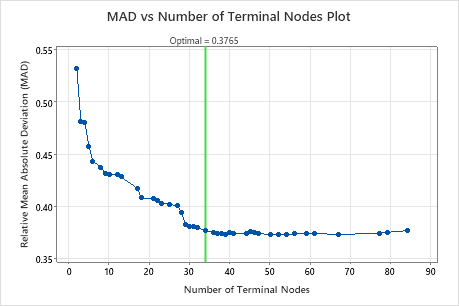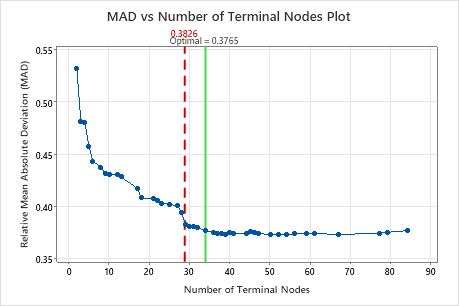# MAD vs number of terminal nodes plot for CART® Regression

Minitab displays a plot of an Mean Absolute Deviation (MAD) values against the number of terminal nodes in the tree so that you can select a tree to evaluate further. If you use a test data set or k-fold cross-validation to validate the performance of the tree, then the MAD value is for the validation data.

The MAD vs number of terminal nodes plot displays the MAD value for each tree. This plot appears when the node splitting method is Least absolute deviation. By default, the initial regression tree is the smallest tree with an MAD value within 1 standard error of the minimum MAD value. When the analysis uses cross-validation or a test data set, the MAD value is from the validation sample. The values for the validation sample typically level off and eventually start to increase as the tree grows larger.

Click Select an Alternative Tree to open an interactive plot that includes a table of model summary statistics. Use the plot to investigate alternative trees with similar performance.

Typically, you select an alternative tree for one of the following two reasons:
• The tree that Minitab selects is part of a pattern where the criterion improves. One or more trees that have a few more nodes are part of the same pattern. Typically, you want to make predictions from a tree with as much prediction accuracy as possible.
• The tree that Minitab selects is part of a pattern where the criterion is relatively flat. One or more trees with similar model summary statistics have much fewer nodes than the optimal tree. Typically, a tree with fewer terminal nodes gives a clearer picture of how each predictor variable affects the response values. A smaller tree also makes it easier to identify a few target groups for further studies. If the difference in prediction accuracy for a smaller tree is negligible, you can also use the smaller tree to evaluate the relationships between the response and the predictor variables

## InterpretationKey Result: MAD vs Number of Terminal Nodes Plot for Tree with 34 Terminal NodesThe regression tree with 34 terminal nodes has an MAD value of approximately 0.38. This tree has the label "Optimal" because the criterion for the creation of the tree was the smallest tree with a deviation value within 1 standard error of the least absolute deviation value. Because this chart shows that the MAD values are relatively stable between trees with about 30 nodes to trees with about 80 nodes, the researchers want to look at the performance of some of the even smaller trees that are similar to the tree in the results. Compare the next graph to see results for a tree with 29 nodes.Key Result: MAD vs Number of Terminal Nodes Plot for Tree with 29 Terminal NodesThe regression tree with 29 terminal nodes has an MAD value of 0.3826. The tree from the initial results keeps the label "Optimal" when you use Select an Alternative Tree to create results for a different tree.
By using this site you agree to the use of cookies for analytics and personalized content.  Read our policy